# Complex Exercises

Michael Fowler

1. Show where in the complex plane are: 1, i,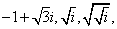and write all these numbers in the form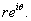2.  State the rule for multiplying two complex numbers of the form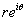, and from that figure out the inverse of a complex number: that is, express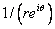as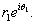3. Find how to invert a number in the other notation: if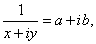find a, b in terms of x, y.

Hint: it helps to multiply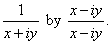4. Show on a diagram where in the complex plane is a cube root of -1, we’ll call itHow many cube roots does -1 have?  Show all possibilities on the diagram.  Next, what about cube roots of 1?  Show them on another figure.  (Note: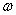is commonly used for a cube root of -1. we also use it, of course, for angular frequency.  Take care not to confuse the two.)

5. Draw a complex number z as a vector (pointing from the origin to z), then draw on the same diagram as vectors  iz, z/i,(being the cube root of -1.)

6. Using, from, deduce the formulas for7. Suppose the point z moves in the complex plane is such a way that at time t, where A is real and.  Where is z at t = 0? Where at t = 1 second? Where at t = 0.5 seconds? Where at t = 0.25 seconds?  Describe how z moves as time progresses.

How would your answer change if A were pure imaginary instead of real?

8. Consider again,.  Differentiate both sides to find an expression for the velocity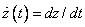as the point moves along its path.  How does the velocity vector relate to the position vector?  Next, find by differentiating again the acceleration vector, and comment on its direction.

9. State briefly how z behaves in time if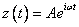for real.  How would this behavior change ifhad a small imaginary part,, where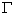is small?  Sketch how z would move in the complex plane, both forpositive andnegative.

10.  Consider the quadratic equation.  For b = 1, both roots equal 1.  Sketch (in the complex plane) how the larger root moves as b varies from 1.2 down through 1 to 0.8.

When you’ve done that, do the same for the other root, preferably in a different color.